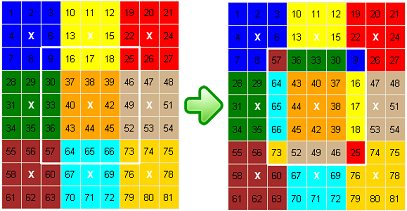HomePuzzle ContestSingle Shift PuzzleFlip Flat RubikDouble Shift PuzzleRotate 3x3 PuzzleRotate 5x5 PuzzleRotate 7x7 PuzzleNine Faces RubikSix Faces Rubik24 Faces Magic Cube4D Rubik CubeQuotes By AuthorQuotes By SubjectQuote Of The DayDetective MysteryReversiLogic TestMath TestWord Of The DayUnsolved ProblemsHall of FameContact UsLoginSign UpSign OutMy AccountChange PasswordAbout UsContributorsLink To UsPrivacy PolicyTerms of ServiceSite Map

## Flat Rubik - Rotate 5x5 Puzzle

Rotate 5x5 Puzzle is a two dimensional variation of Rubik's Cube that consists of 3x3 color bocks. For the puzzle to be solved, colors should be separated and each block must be a solid color (see the left image in Figure 1).
There are 9 square marked with white “X”. By clicking on the white “X” square, you can rotate a 5x5 square block around the “X” 90 degrees. There are two options: 'Rotate 5x5 Square Clockwise' and 'Rotate 5x5 Square Counterclockwise'.Figure 1
For example, if you select the 'Rotate 5x5 Square Clockwise' option and click on white “X” square between squares #40 and #42 (an intersection of column #5 and row #5) a 5x5 square block around white “X” square will rotate clockwise 90 degrees (see Figure 1). The 5x5 square block includes all squares between (and including) row #3 and row #7 and between (and including) column #3 and column #7 (two rows up and two rows down, and two columns left and two columns right from the white “X” square).

Columns are enumerated from #1 to #9. Next column after column #9 is a column #1 again. The previous column for column #1 is column #9. The same rule applies to the rows.Figure 2
For example, if you select the 'Rotate 5x5 Square Clockwise' option and click on white “X” square between square #4 and #6 (an intersection of column #2 and row #2) a 5x5 square block around white “X” square will rotate clockwise 90 degrees (see Figure 2). The 5x5 square block includes all square two rows up and two rows down and, two columns left and two columns right from the white “X” square. It includes 4x4 square between the row #1 and row #4 and between column #1 and column #4, and also squares 61, 62, 63, 70, and 81 from row #9, and squares from 21, 24, 27, and 48 from column #9.